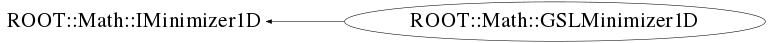# class ROOT::Math::GSLMinimizer1D: public ROOT::Math::IMinimizer1D

```

Minimizer for arbitrary one dimensional functions.

Implemented using GSL, for detailed description see:
<A HREF="http://www.gnu.org/software/gsl/manual/html_node/One-dimensional-Minimization.html">GSL online doc</A>

The algorithms uspported are only bracketing algorithm which do not use derivatives information.
The algorithms which can be chosen at construction time are  GOLDENSECTION, whic is the simplest method
but the slowest and BRENT (the default one) which combines the golden section with a parabolic interpolation.

This class does not support copying
@ingroup Min1D

```

## Function Members (Methods)

public:
 virtual ~GSLMinimizer1D() virtual double FValLower() const virtual double FValMinimum() const virtual double FValUpper() const ROOT::Math::GSLMinimizer1D GSLMinimizer1D(ROOT::Math::Minim1D::Type type = Minim1D::kBRENT) ROOT::Math::IMinimizer1D ROOT::Math::IMinimizer1D::IMinimizer1D() ROOT::Math::IMinimizer1D ROOT::Math::IMinimizer1D::IMinimizer1D(const ROOT::Math::IMinimizer1D&) int Iterate() virtual int Iterations() const virtual bool Minimize(int maxIter, double absTol, double relTol) virtual const char* Name() const void SetFunction(ROOT::Math::GSLFuncPointer f, void* params, double xmin, double xlow, double xup) virtual int Status() const static int TestInterval(double xlow, double xup, double epsAbs, double epsRel) virtual double XLower() const virtual double XMinimum() const virtual double XUpper() const
private:
 ROOT::Math::GSLMinimizer1D GSLMinimizer1D(const ROOT::Math::GSLMinimizer1D&) ROOT::Math::GSLMinimizer1D& operator=(const ROOT::Math::GSLMinimizer1D&)

## Data Members

private:
 ROOT::Math::GSLFunctionWrapper* fFunction bool fIsSet int fIter double fLow double fMin ROOT::Math::GSL1DMinimizerWrapper* fMinimizer int fStatus status of last minimization (==0 ok =1 failed) double fUp double fXlow double fXmin double fXup

## Class Charts## Function documentation

explicit GSLMinimizer1D(Minim1D::Type type=Minim1D::kBRENT)
```         Construct the minimizer passing the minimizer type using the Minim1D::Algorithm enumeration

```
virtual ~GSLMinimizer1D()
```         Destructor: free allocated resources

```
GSLMinimizer1D(const GSLMinimizer1D &)
``` usually copying is non trivial, so we make this unaccessible
```
void SetFunction( const UserFunc & f, double xmin, double xlow, double xup)
```          Set, or reset, minimizer to use the function f and the initial search interval [xlow, xup], with a guess for the location of the minimum xmin.
The condition : \f\$ f(xlow) > f(xmin) < f(xup)\f\$  must be satisfied

```
int Iterate()
```          Perform a minimizer iteration and
if an unexepcted problem occurr then an error code will be returned

```
double XMinimum() const
```          Return current estimate of the position of the minimum

```
double XLower() const
```         Return current lower bound of the minimization interval

```
double XUpper() const
```         Return current upper bound of the minimization interval

```
double FValMinimum() const
```          Return function value at current estimate of the minimum

```
double FValLower() const
```         Return function value at current lower bound of the minimization interval

```
double FValUpper() const
```         Return function value at current upper bound of the minimization interval

```
bool Minimize(int maxIter, double absTol, double relTol)
```         Find minimum position iterating until convergence specified by the absolute and relative tolerance or
the maximum number of iteration is reached
Return true is result is successfull
\@param maxIter maximum number of iteration
\@param absTol desired absolute error in the minimum position
\@param absTol desired relative error in the minimum position

```
int Iterations() const
```         Return number of iteration used to find minimum

```
int Status() const
```         Return status of last minimization

```
`{ return fStatus; }`
const char * Name() const
```         Return name of minimization algorithm

```
int TestInterval(double xlow, double xup, double epsAbs, double epsRel)
```         Test convergence of the interval.
The test returns success if
\f[
|x_{min}-x_{truemin}| < epsAbs + epsRel *x_{truemin}
\f]

```